# Selina Solutions Concise Maths Class 10 Chapter 22 Heights and Distances Exercise 22(C)

Students will acquire a complete picture in solving these exercise problems. Different kinds of models are discussed to provide a strong conceptual knowledge over the topic. For further guidance, students can refer to the Selina Solutions for Class 10 Maths prepared by subject experts. The Concise Selina Solutions for Class 10 Maths Chapter 22 Heights and Distances Exercise 22(C) solution PDF can be effortlessly downloaded from the link given below.

## Selina Solutions Concise Maths Class 10 Chapter 22 Heights and Distances Exercise 22(C) Download PDF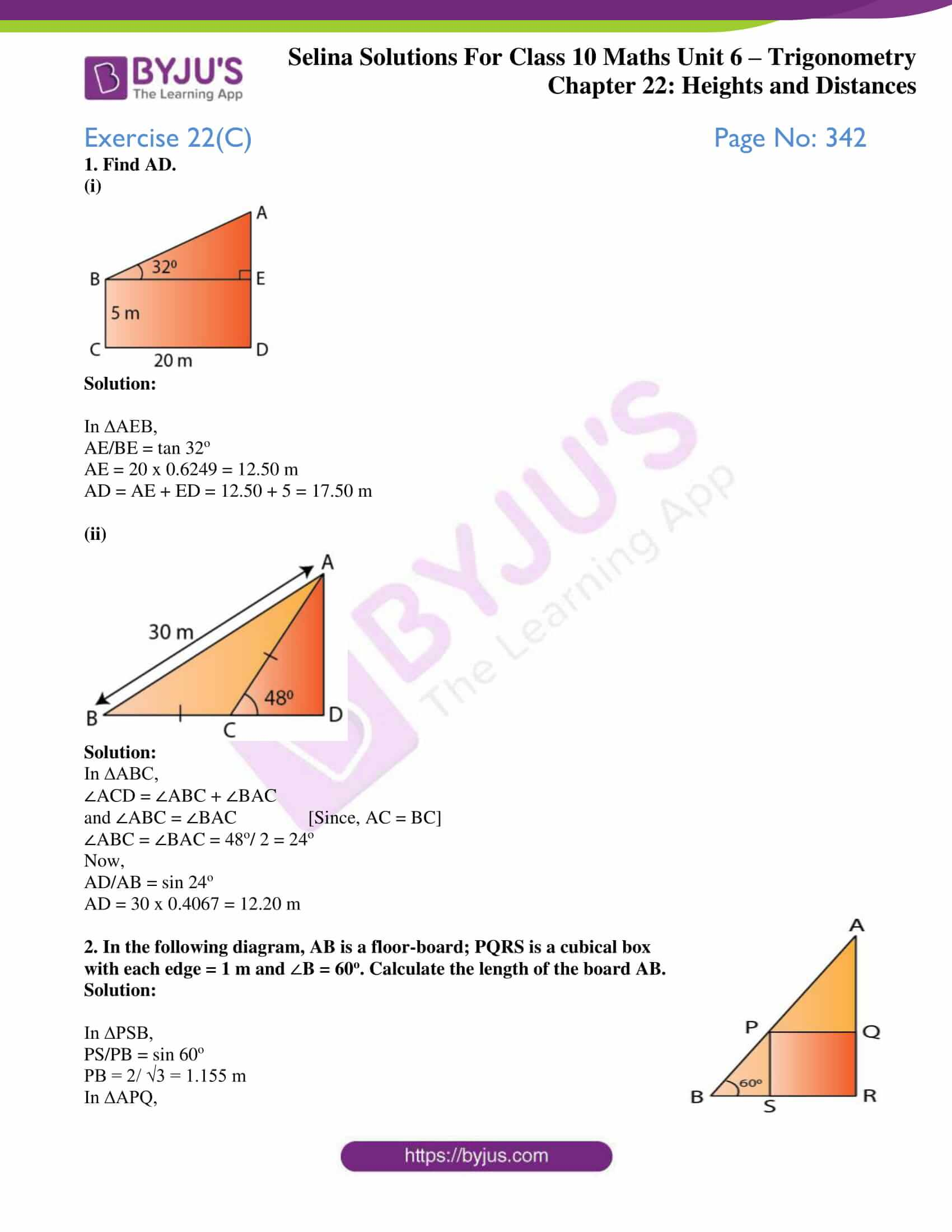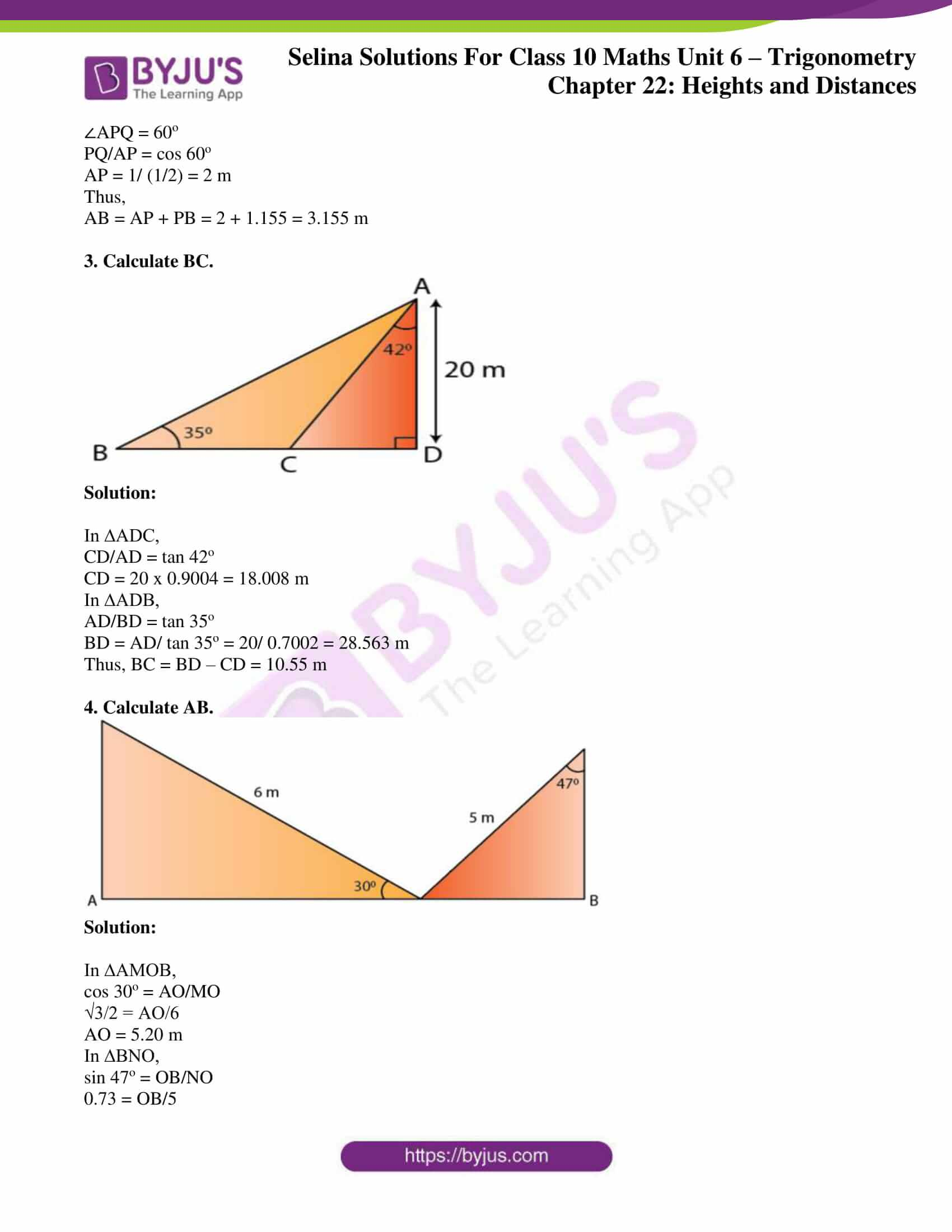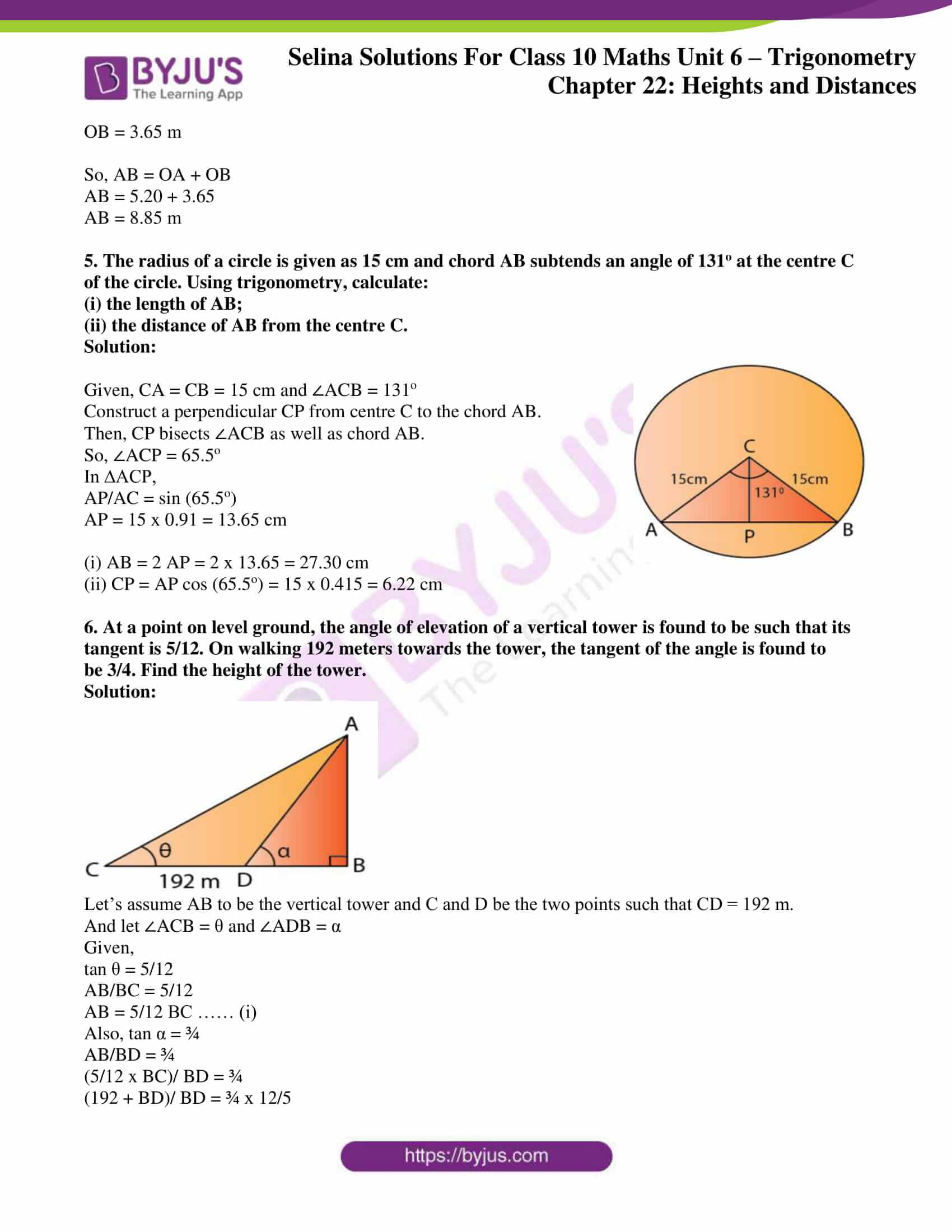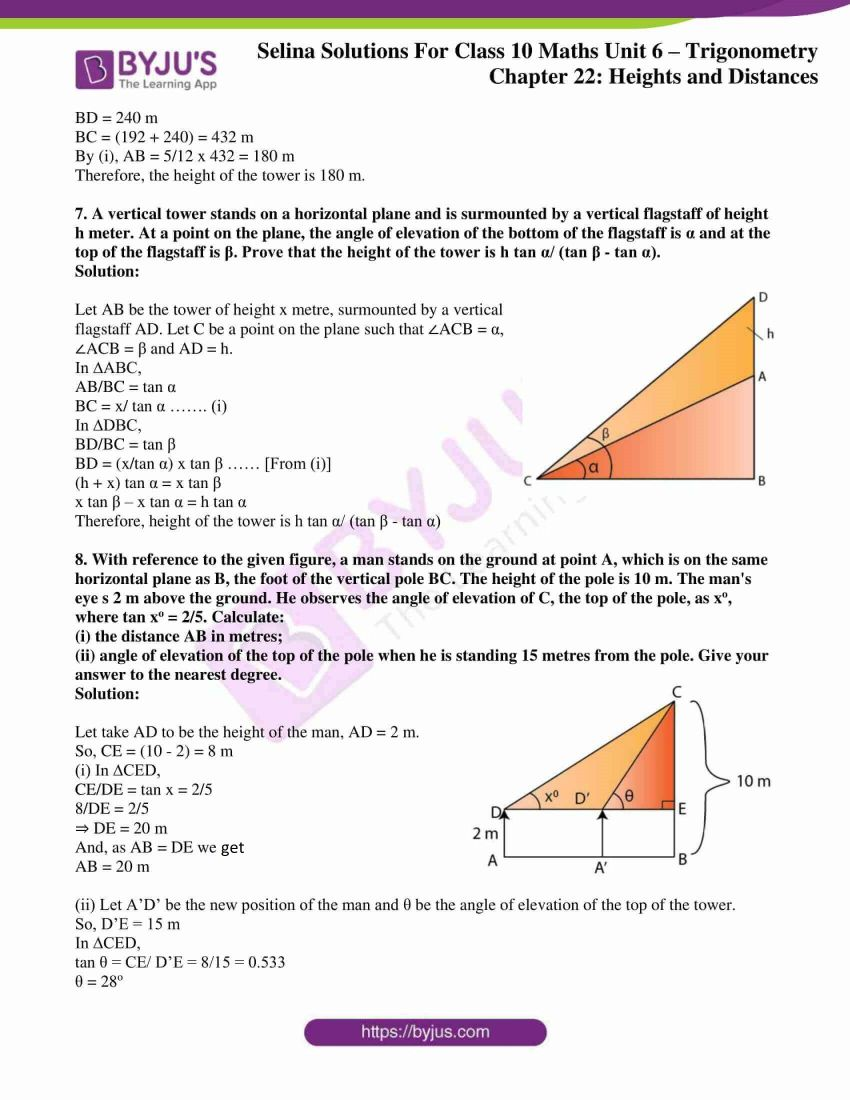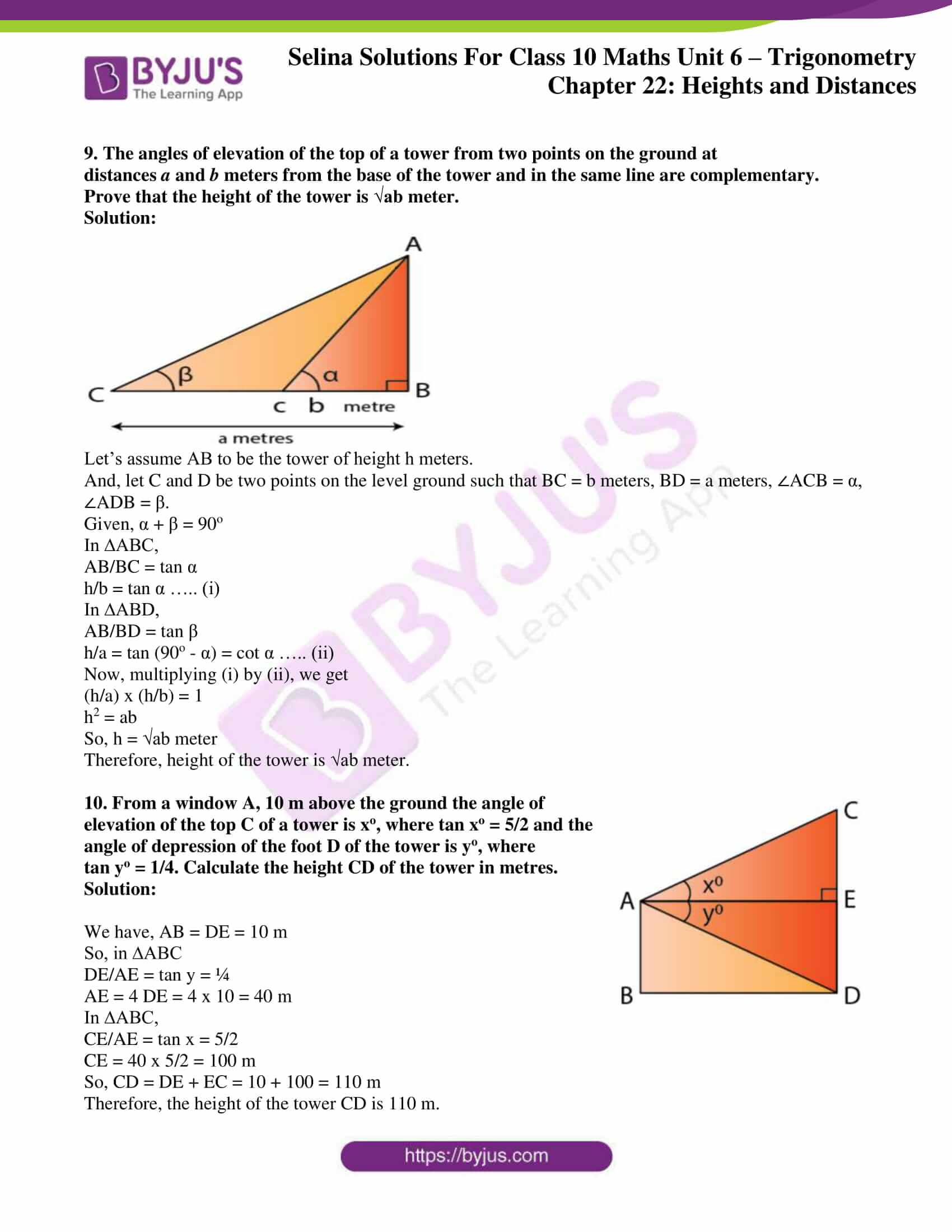### Access other exercises of Selina Solutions Concise Maths Class 10 Chapter 22 Heights and Distances

Exercise 22(A) Solutions

Exercise 22(B) Solutions

### Access Selina Solutions Concise Maths Class 10 Chapter 22 Heights and Distances Exercise 22(C)

(i)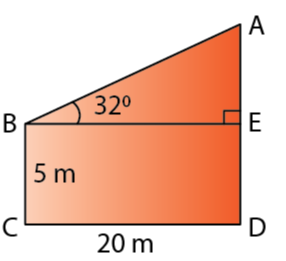Solution:

In ∆AEB,

AE/BE = tan 32o

AE = 20 x 0.6249 = 12.50 m

AD = AE + ED = 12.50 + 5 = 17.50 m

(ii)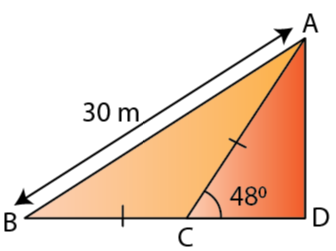Solution:

In ∆ABC,

∠ACD = ∠ABC + ∠BAC

and ∠ABC = ∠BAC [Since, AC = BC]

∠ABC = ∠BAC = 48o/ 2 = 24o

Now,

AD = 30 x 0.4067 = 12.20 m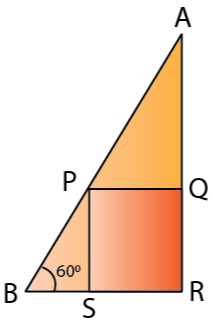2. In the following diagram, AB is a floor-board; PQRS is a cubical box with each edge = 1 m and B = 60o. Calculate the length of the board AB.

Solution:

In ∆PSB,

PS/PB = sin 60o

PB = 2/ √3 = 1.155 m

In ∆APQ,

∠APQ = 60o

PQ/AP = cos 60o

AP = 1/ (1/2) = 2 m

Thus,

AB = AP + PB = 2 + 1.155 = 3.155 m

3. Calculate BC.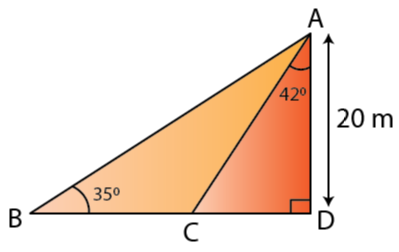Solution:

CD = 20 x 0.9004 = 18.008 m

BD = AD/ tan 35o = 20/ 0.7002 = 28.563 m

Thus, BC = BD – CD = 10.55 m

4. Calculate AB.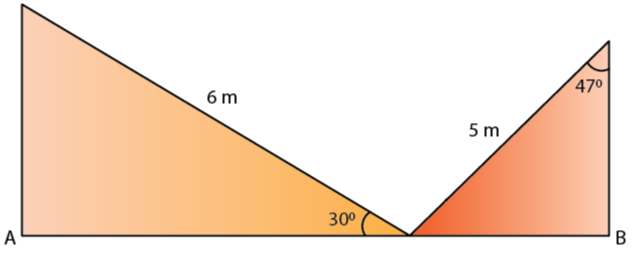Solution:

In ∆AMOB,

cos 30o = AO/MO

√3/2 = AO/6

AO = 5.20 m

In ∆BNO,

sin 47o = OB/NO

0.73 = OB/5

OB = 3.65 m

So, AB = OA + OB

AB = 5.20 + 3.65

AB = 8.85 m

5. The radius of a circle is given as 15 cm and chord AB subtends an angle of 131o at the centre C of the circle. Using trigonometry, calculate:

(i) the length of AB;

(ii) the distance of AB from the centre C.

Solution: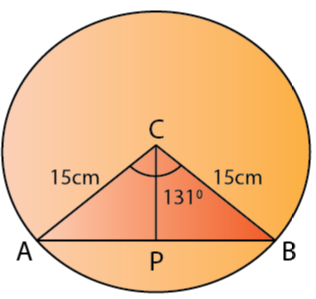Given, CA = CB = 15 cm and ∠ACB = 131o

Construct a perpendicular CP from centre C to the chord AB.

Then, CP bisects ∠ACB as well as chord AB.

So, ∠ACP = 65.5o

In ∆ACP,

AP/AC = sin (65.5o)

AP = 15 x 0.91 = 13.65 cm

(i) AB = 2 AP = 2 x 13.65 = 27.30 cm

(ii) CP = AP cos (65.5o) = 15 x 0.415 = 6.22 cm

6. At a point on level ground, the angle of elevation of a vertical tower is found to be such that its tangent is 5/12. On walking 192 meters towards the tower, the tangent of the angle is found to be 3/4. Find the height of the tower.

Solution: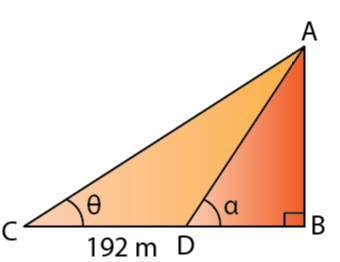Let’s assume AB to be the vertical tower and C and D be the two points such that CD = 192 m.

And let ∠ACB = θ and ∠ADB = α

Given,

tan θ = 5/12

AB/BC = 5/12

AB = 5/12 BC …… (i)

Also, tan α = ¾

AB/BD = ¾

(5/12 x BC)/ BD = ¾

(192 + BD)/ BD = ¾ x 12/5

BD = 240 m

BC = (192 + 240) = 432 m

By (i), AB = 5/12 x 432 = 180 m

Therefore, the height of the tower is 180 m.

7. A vertical tower stands on a horizontal plane and is surmounted by a vertical flagstaff of height h meter. At a point on the plane, the angle of elevation of the bottom of the flagstaff is α and at the top of the flagstaff is β. Prove that the height of the tower is h tan α/ (tan β – tan α).

Solution: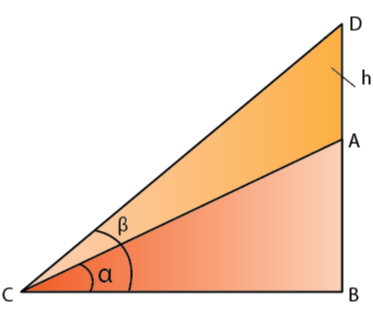Let AB be the tower of height x metre, surmounted by a vertical flagstaff AD. Let C be a point on the plane such that ∠ACB = α, ∠ACB = β and AD = h.

In ∆ABC,

AB/BC = tan α

BC = x/ tan α ……. (i)

In ∆DBC,

BD/BC = tan β

BD = (x/tan α) x tan β …… [From (i)]

(h + x) tan α = x tan β

x tan β – x tan α = h tan α

Therefore, height of the tower is h tan α/ (tan β – tan α)

8. With reference to the given figure, a man stands on the ground at point A, which is on the same horizontal plane as B, the foot of the vertical pole BC. The height of the pole is 10 m. The man’s eye s 2 m above the ground. He observes the angle of elevation of C, the top of the pole, as xo, where tan xo = 2/5. Calculate:

(i) the distance AB in metres;

(ii) angle of elevation of the top of the pole when he is standing 15 metres from the pole. Give your answer to the nearest degree.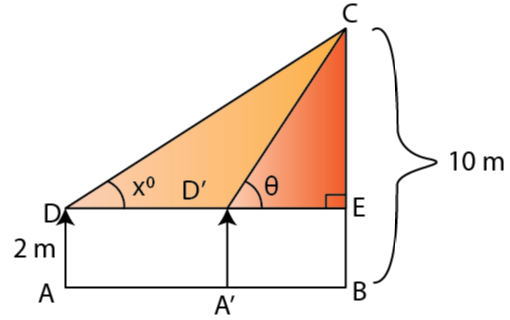Solution:

Let take AD to be the height of the man, AD = 2 m.

So, CE = (10 – 2) = 8 m

(i) In ∆CED,

CE/DE = tan x = 2/5

8/DE = 2/5

⇒ DE = 20 m

And, as AB = DE we get

AB = 20 m

(ii) Let A’D’ be the new position of the man and θ be the angle of elevation of the top of the tower.

So, D’E = 15 m

In ∆CED,

tan θ = CE/ D’E = 8/15 = 0.533

θ = 28o

9. The angles of elevation of the top of a tower from two points on the ground at distances a and b meters from the base of the tower and in the same line are complementary. Prove that the height of the tower is √ab meter.

Solution: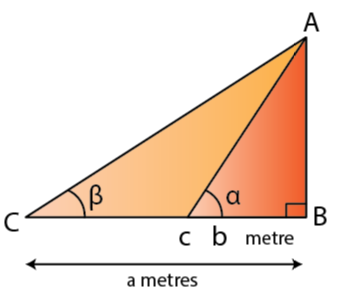Let’s assume AB to be the tower of height h meters.

And, let C and D be two points on the level ground such that BC = b meters, BD = a meters, ∠ACB = α, ∠ADB = β.

Given, α + β = 90o

In ∆ABC,

AB/BC = tan α

h/b = tan α ….. (i)

In ∆ABD,

AB/BD = tan β

h/a = tan (90o – α) = cot α ….. (ii)

Now, multiplying (i) by (ii), we get

(h/a) x (h/b) = 1

h2 = ab

So, h = √ab meter

Therefore, height of the tower is √ab meter.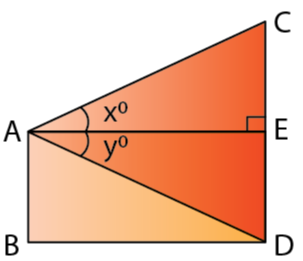10. From a window A, 10 m above the ground the angle of elevation of the top C of a tower is xo, where tan xo = 5/2 and the angle of depression of the foot D of the tower is yo, where tan yo = 1/4. Calculate the height CD of the tower in metres.

Solution:

We have, AB = DE = 10 m

So, in ∆ABC

DE/AE = tan y = ¼

AE = 4 DE = 4 x 10 = 40 m

In ∆ABC,

CE/AE = tan x = 5/2

CE = 40 x 5/2 = 100 m

So, CD = DE + EC = 10 + 100 = 110 m

Therefore, the height of the tower CD is 110 m.